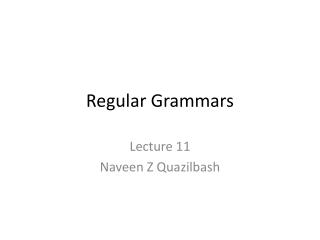# Regular Grammars - PowerPoint PPT PresentationDownload PresentationRegular Grammars

Regular Grammars
Download Presentation## Regular Grammars

- - - - - - - - - - - - - - - - - - - - - - - - - - - E N D - - - - - - - - - - - - - - - - - - - - - - - - - - -
##### Presentation Transcript

1. Regular Grammars Lecture 11 Naveen Z Quazilbash

2. Overview • Examples • Definition • Language defined by the grammar • Linearity of grammar • Equivalence with NFAs

3. Examples • Example 1: S aAb AcA Aε • The corresponding language is ac*b • Example 2: S aA AbB BaA Bε • The corresponding language is (ab)+ S=>aAb=>acAb=>accAb=>accb S=>aAb=>ab S=>aA=>abB=>ab S=>aA=>abB=>abaA=>ababB=>abab

4. Some Definitions • The substitution rules that form a grammar are called “Productions”. • The left side of a rule is a “variable”. • The right side is a mixture of variables and symbols of the alphabet (a.k.a “Terminal symbols”).

5. Some Definitions Contd. (2) • A grammar defines the language of all strings that can be derived from the “Start variable, S”, by successive applications of productions. • Note that the strings of this language do not contain variables.

6. Some Definitions Contd. (3) • A sequence of substitutions that leads from S to some string of the language is called a “Derivation”. • Example: • S=>aA=>abB=>ab is a derivation; we may write it in a shorter form as S=>*ab, • where “=>*” means “several steps”.

7. Formal Definition of Grammar • Formally, a grammar is a four tuple: G= {V, T, S, P}, where • V= set of variables, e.g. V={S, A, B} • T= set of terminal symbols, e.g. T={a, b} • S= start variable (analogous to the start state in automaton). • P= set of productions, e.g. P={SaA, AbB, BaA, Bε} • Note that V and T must be non-empty and disjoint.

8. Language defined by the Grammar • The set of all strings derivable from S is the “language defined (or generated) by the grammar”.

9. Linearity of Grammar • A Grammar is “Linear” if all productions are of the form Aw1Bw2 or Aw3, where A and B are variables and w1,w2 and w3 are strings (with no variables in them). • Example of a linear grammar: SaB Sε BSb • Shorter notation: Sab | ε • Language: L={anbn: n≥0}, (not a regular language) • Thus, a linear grammar may generate a language that is not accepted by any finite automaton. S=>ε S=>aB=>aSb=>ab S=>aB=>aSb=>aaBb=>aaSbb=>aabb

10. NON-LINEAR ???

11. Linearity of Grammar-Contd.. • Right linear grammar: VT*V VT* • Left linear grammar: VVT* VT*

12. ASSIGNMENT NO.2 • For each of the following three languages on ∑={a,b}, draw a DFA that accepts it: (6 points) • All strings that have no b’s (note that it includes ε). • All strings with atleast two a’s and any number of b’s. • All strings with atmost two a’s and any number of b’s. • Due Date: 28th March 2013.